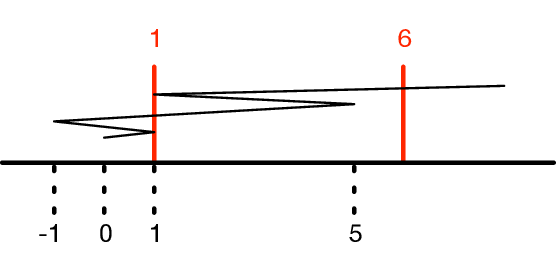시간 제한 메모리 제한 제출 정답 맞은 사람 정답 비율
5 초 1024 MB 4 4 4 100.000%

## 문제

To make a paper snowflake, you fold a sheet of paper in various places and then cut some parts out of the folded sheet. When you unfold the sheet, it can make a very nice pattern. That is, if the folds and cuts are chosen well.

Samantha has recently taken up this hobby but with a "modern art" twist. To get started, she explores folding a strip of paper that is $L$ picometres long (Samantha likes to measure her folds and cuts very precisely). She places it on the real line with one end at $0$ and the other at $L$. The left end at point $0$ is affixed to this point, the other endpoint at position $L$ is the loose endpoint.

She then performs a series of folds. For the first fold, Samantha creases the paper $f_1$ picometres from the loose end and folds the loose end over this fold. The loose end is now pointing towards the left. For the second fold, she then creases the top portion of the paper $f_2$ picometres from the loose end ($f_2 < f_1$) and folds the top portion of the paper over this point back to the right over this crease point. The loose end is now pointing towards the right.

She alternates folding back-and-forth this way. At each step, she creases the top portion of the paper $f_i$ picometres from the loose end ($f_i < f_{i-1}$) and folds the loose end over the crease.

She will now cut the paper at $M$ distinct locations, which will split the paper into $M+1$ piles. Each of the piles will have zero or more layers of paper in it. The figure below depicts the folded paper from the first sample input, the cuts (solid lines), and the $x$-locations of where the paper was folded (dashed lines).

What is the total length of paper in each of the $M+1$ piles?## 입력

The first line of input consists of three integers $N$ ($1 \leq N \leq 10^5$), which is the number of folds, $M$ ($1 \leq M \leq 10^5$), which is the number of cuts, and $L$ ($2 \leq L \leq 10^{18}$), which is the length of the paper in picometres.

The second line describes the folds. This line contains $N$ integers $f_1, f_2, \ldots, f_N$, indicating that the $i^\textrm{th}$ fold occurred $f_i$ picometres from the loose end. These values satisfy $1 \leq f_N < f_{N-1} < \cdots < f_1 < L$.

The third line describes the cuts. This line contains $M$ integers $c_1, c_2, \ldots, c_M$, indicating that the $i^\textrm{th}$ cut is $c_i$ picometres from the affixed point. These values satisfy $-10^{18} \leq c_1 < c_2 < \cdots < c_M \leq 10^{18}$.

## 출력

Output the total length of paper in each of the $M+1$ piles, in order from left to right.

## 예제 입력 1

4 2 20
19 17 11 7
1 6


## 예제 출력 1

5 13 2


## 예제 입력 2

1 1 10
9
0


## 예제 출력 2

8 2


## 예제 입력 3

1 2 1000000000000000000
500000000000000000
0 500000000000000000


## 예제 출력 3

0 1000000000000000000 0


## 출처• 문제를 만든 사람: Zachary Friggstad